International
Tables for
Crystallography
Volume B
Reciprocal space
Edited by U. Shmueli

International Tables for Crystallography (2006). Vol. B, ch. 1.3, p. 33   | 1 | 2 |

Section 1.3.2.3.9.5. Transformation of coordinates

G. Bricognea

aMRC Laboratory of Molecular Biology, Hills Road, Cambridge CB2 2QH, England, and LURE, Bâtiment 209D, Université Paris-Sud, 91405 Orsay, France

1.3.2.3.9.5. Transformation of coordinates

| top | pdf |

Let σ be a smooth non-singular change of variables in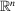, i.e. an infinitely differentiable mapping from an open subset Ω ofto Ω′ in, whose Jacobian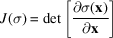vanishes nowhere in Ω. By the implicit function theorem, the inverse mappingfrom Ω′ to Ω is well defined.

If f is a locally summable function on Ω, then the function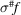defined by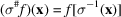is a locally summable function on Ω′, and for any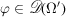we may write: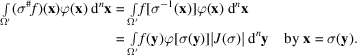In terms of the associated distributions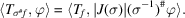This operation can be extended to an arbitrary distribution T by defining its image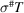under coordinate transformation σ through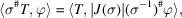which is well defined provided that σ is proper, i.e. that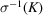is compact whenever K is compact.

For instance, if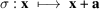is a translation by a vector a in, then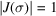;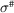is denoted by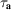, and the translate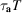of a distribution T is defined by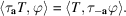Let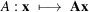be a linear transformation defined by a non-singular matrix A. Then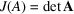, and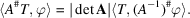This formula will be shown later (Sections 1.3.2.6.5, 1.3.4.2.1.1) to be the basis for the definition of the reciprocal lattice.

In particular, if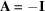, where I is the identity matrix, A is an inversion through a centre of symmetry at the origin, and denoting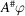by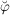we have: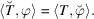T is called an even distribution if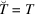, an odd distribution if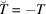.

If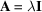with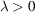, A is called a dilation and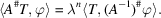Writing symbolically δ as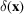and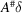as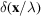, we have: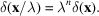If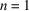and f is a function with isolated simple zeros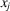, then in the same symbolic notation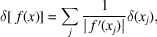where each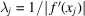is analogous to a `Lorentz factor' at zero.